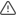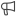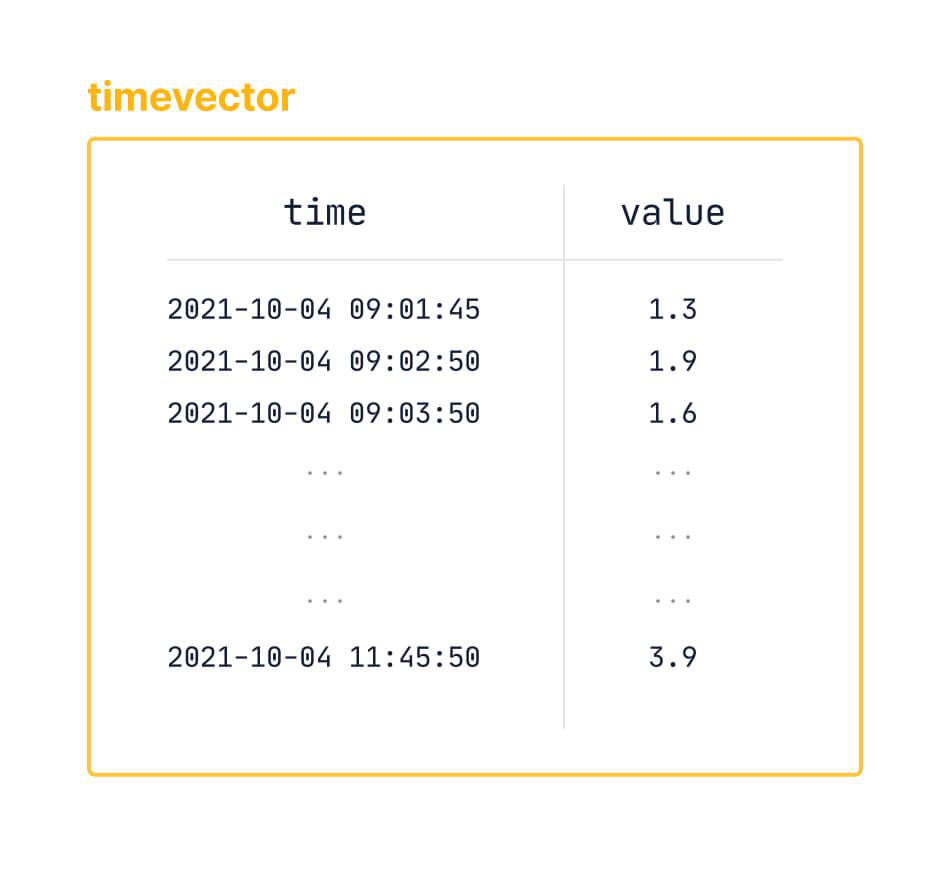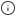Function pipelines are an experimental feature, designed to radically improve how you write queries to analyze data in PostgreSQL and SQL. They work by applying principles from functional programming and popular tools like Python Pandas, and PromQL.###### Warning

Experimental features could have bugs. They might not be backwards compatible, and could be removed in future releases. Use these features at your own risk, and do not use any experimental features in production.###### Important

The `timevector()` function materializes all its data points in memory. This means that if you use it on a very large dataset, it runs out of memory. Do not use the `timevector` function on a large dataset, or in production.

SQL is the best language for data analysis, but it is not perfect, and at times it can be difficult to construct the query you want. For example, this query gets data from the last day from the measurements table, sorts the data by the time column, calculates the delta between the values, takes the absolute value of the delta, and then takes the sum of the result of the previous steps:

`SELECT device id,sum(abs_delta) as volatilityFROM ( SELECT device_id,abs(val - lag(val) OVER last_day) as abs_deltaFROM measurementsWHERE ts >= now()-'1 day'::interval) calc_deltaGROUP BY device_id;`

You can express the same query with a function pipeline like this:

`SELECT device_id,    toolkit_experimental.timevector(ts, val)        -> toolkit_experimental.sort()        -> toolkit_experimental.delta()        -> toolkit_experimental.abs()        -> toolkit_experimental.sum() as volatilityFROM measurementsWHERE ts >= now()-'1 day'::intervalGROUP BY device_id;`

Function pipelines are completely SQL compliant, meaning that any tool that speaks SQL is able to support data analysis using function pipelines.

## Anatomy of a function pipeline

Function pipelines are built as a series of elements that work together to create your query. The most important part of a pipeline is a custom data type called a `timevector`. The other elements then work on the `timevector` to build your query, using a custom operator to define the order in which the elements are run.

### Timevectors

A `timevector` is a collection of time,value pairs with a defined start and end time, that could something like this:Your entire database might have time,value pairs that go well into the past and continue into the future, but the `timevector` has a defined start and end time within that dataset, which could look something like this:To construct a `timevector` from your data, use a custom aggregate and pass in the columns to become the time,value pairs. It uses a `WHERE` clause to define the limits of the subset, and a `GROUP BY` clause to provide identifying information about the time-series. For example, to construct a `timevector` from a dataset that contains temperatures, the SQL looks like this:

`SELECT device_id, toolkit_experimental.timevector(ts, val)FROM measurementsWHERE ts >= now() - '1 day'::intervalGROUP BY device_id;`

### Custom operator

Function pipelines use a single custom operator of `->`. This operator is used to apply and compose multiple functions. The `->` operator takes the inputs on the left of the operator, and applies the operation on the right of the operator. To put it more plainly, you can think of it as "do the next thing."

A typical function pipeline could look something like this:

`SELECT device_id,  toolkit_experimental.timevector(ts, val)        -> toolkit_experimental.sort()        -> toolkit_experimental.delta()        -> toolkit_experimental.abs()        -> toolkit_experimental.sum() as volatilityFROM measurementsWHERE ts >= now() - '1 day'::intervalGROUP BY device_id;`

While it might look at first glance as though `timevector(ts, val)` operation is an argument to `sort()`, in a pipeline these are all regular function calls. Each of the calls can only operate on the things in their own parentheses, and don't know about anything to the left of them in the statement.

Each of the functions in a pipeline returns a custom type that describes the function and its arguments, these are all pipeline elements. The `->` operator performs one of two different types of actions depending on the types on its right and left sides:

• Applies a pipeline element to the left hand argument: performing the function described by the pipeline element on the incoming data type directly.
• Compose pipeline elements into a combined element that can be applied at some point in the future. This is an optimization that allows you to nest elements to reduce the number of passes that are required.

The operator determines the action to perform based on its left and right arguments.

### Pipeline elements

There are two main types of pipeline elements:

• Transforms change the contents of the `timevector`, returning the updated vector.
• Finalizers finish the pipeline and output the resulting data.

Transform elements take in a `timevector` and produce a `timevector`. They are the simplest element to compose, because they produce the same type. For example:

`SELECT device_id, toolkit_experimental.timevector(ts, val)     -> toolkit_experimental.sort()        -> toolkit_experimental.delta()        -> toolkit_experimental.map(\$\$ (\$value^3 + \$value^2 + \$value * 2) \$\$)        -> toolkit_experimental.lttb(100)FROM measurements`

Finalizer elements end the `timevector` portion of a pipeline. They can produce an output in a specified format. or they can produce an aggregate of the `timevector`.

For example, a finalizer element that produces an output:

`SELECT device_id, toolkit_experimental.timevector(ts, val)    -> toolkit_experimental.sort()    -> toolkit_experimental.delta()    -> toolkit_experimental.unnest()FROM measurements`

Or a finalizer element that produces an aggregate:

`SELECT device_id, toolkit_experimental.timevector(ts, val)    -> toolkit_experimental.sort()    -> toolkit_experimental.delta()    -> toolkit_experimental.time_weight()FROM measurements`

The third type of pipeline elements are aggregate accessors and mutators. These work on a `timevector` in a pipeline, but they also work in regular aggregate queries. An example of using these in a pipeline:

`SELECT percentile_agg(val) -> toolkit_experimental.approx_percentile(0.5)FROM measurements`

## Transform elements

Transform elements take a `timevector`, and produce a `timevector`.

### Vectorized math functions

Vectorized math function elements modify each `value` inside the `timevector` with the specified mathematical function. They are applied point-by-point and they produce a one-to-one mapping from the input to output `timevector`. Each point in the input has a corresponding point in the output, with its `value` transformed by the mathematical function specified.

Elements are always applied left to right, so the order of operations is not taken into account even in the presence of explicit parentheses. This means for a `timevector` row `('2020-01-01 00:00:00+00', 20.0)`, this pipeline works:

`timevector('2021-01-01 UTC', 10) -> add(5) -> (mul(2) -> add(1))`

And this pipeline works in the same way:

`timevector('2021-01-01 UTC', 10) -> add(5) -> mul(2) -> add(1)`

Both of these examples produce `('2020-01-01 00:00:00+00', 31.0)`.

If multiple arithmetic operations are needed and precedence is important, consider using a Lambda instead.

### Unary mathematical functions

Unary mathematical function elements apply the corresponding mathematical function to each datapoint in the `timevector`, leaving the timestamp and ordering the same. The available elements are:

ElementDescription
`abs()`Computes the absolute value of each value
`cbrt()`Computes the cube root of each value
`ceil()`Computes the first integer greater than or equal to each value
`floor()`Computes the first integer less than or equal to each value
`ln()`Computes the natural logarithm of each value
`log10()`Computes the base 10 logarithm of each value
`round()`Computes the closest integer to each value
`sign()`Computes +/-1 for each positive/negative value
`sqrt()`Computes the square root for each value
`trunc()`Computes only the integer portion of each value

Even if an element logically computes an integer, `timevectors` only deal with double precision floating point values, so the computed value is the floating point representation of the integer. For example:

`-- NOTE: the (pipeline -> unnest()).* allows for time, value columns to be produced without a subselectSELECT (    toolkit_experimental.timevector(time, value)    -> toolkit_experimental.abs()    -> toolkit_experimental.unnest()).*FROM (VALUES (TimestampTZ '2021-01-06 UTC',   0.0 ),             (            '2021-01-01 UTC',  25.0 ),             (            '2021-01-02 UTC',   0.10),             (            '2021-01-04 UTC', -10.0 ),             (            '2021-01-05 UTC',   3.3 )     ) as v(time, value);`

The output for this example:

`time          | value------------------------+------- 2021-01-06 00:00:00+00 |     0 2021-01-01 00:00:00+00 |    25 2021-01-02 00:00:00+00 |   0.1 2021-01-04 00:00:00+00 |    10 2021-01-05 00:00:00+00 |   3.3(5 rows)`

### Binary mathematical functions

Binary mathematical function elements run the corresponding mathematical function on the `value` in each point in the `timevector`, using the supplied number as the second argument of the function. The available elements are:

ElementDescription
`add(N)`Computes each value plus `N`
`div(N)`Computes each value divided by `N`
`logn(N)`Computes the logarithm base `N` of each value
`mod(N)`Computes the remainder when each number is divided by `N`
`mul(N)`Computes each value multiplied by `N`
`power(N)`Computes each value taken to the `N` power
`sub(N)`Computes each value less `N`

These elements calculate `vector -> power(2)` by squaring all of the `values`, and `vector -> logn(3)` gives the log-base-3 of each `value`. For example:

`SELECT (    toolkit_experimental.timevector(time, value)    -> toolkit_experimental.power(2)    -> toolkit_experimental.unnest()).*FROM (VALUES (TimestampTZ '2021-01-06 UTC',   0.0 ),             (            '2021-01-01 UTC',  25.0 ),             (            '2021-01-02 UTC',   0.10),             (            '2021-01-04 UTC', -10.0 ),             (            '2021-01-05 UTC',   3.3 )     ) as v(time, value);`

The output for this example:

`time          |        value         ------------------------+---------------------- 2021-01-06 00:00:00+00 |                    0 2021-01-01 00:00:00+00 |                  625 2021-01-02 00:00:00+00 | 0.010000000000000002 2021-01-04 00:00:00+00 |                  100 2021-01-05 00:00:00+00 |   10.889999999999999(5 rows)`

### Compound transforms

Mathematical transforms are applied only to the `value` in each point in a `timevector` and always produce one-to-one output `timevectors`. Compound transforms can involve both the `time` and `value` parts of the points in the `timevector`, and they are not necessarily one-to-one. One or more points in the input can be used to produce zero or more points in the output. So, where mathematical transforms always produce `timevectors` of the same length, compound transforms can produce larger or smaller `timevectors` as an output.

#### Delta transforms

A `delta()` transform calculates the difference between consecutive `values` in the `timevector`. The first point in the `timevector` is omitted as there is no previous value and it cannot have a `delta()`. Data should be sorted using the `sort()` element before passing into `delta()`. For example:

`SELECT (    toolkit_experimental.timevector(time, value)    -> toolkit_experimental.sort()    -> toolkit_experimental.delta()    -> toolkit_experimental.unnest()).*FROM (VALUES (TimestampTZ '2021-01-06 UTC',   0.0 ),             (            '2021-01-01 UTC',  25.0 ),             (            '2021-01-02 UTC',   0.10),             (            '2021-01-04 UTC', -10.0 ),             (            '2021-01-05 UTC',   3.3 )     ) as v(time, value);`

The output for this example:

`time          | value------------------------+------- 2021-01-02 00:00:00+00 | -24.9 2021-01-04 00:00:00+00 | -10.1 2021-01-05 00:00:00+00 |  13.3 2021-01-06 00:00:00+00 |  -3.3(4 rows)`###### Note

The first row of the output is missing, as there is no way to compute a delta without a previous value.

#### Fill method transform

The `fill_to()` transform ensures that there is a point at least every `interval`, if there is not a point, it fills in the point using the method provided. The `timevector` must be sorted before calling `fill_to()`. The available fill methods are:

fill_methoddescription
LOCFLast object carried forward, fill with last known value prior to the hole
InterpolateFill the hole using a collinear point with the first known value on either side
LinearThis is an alias for interpolate
NearestFill with the matching value from the closer of the points preceding or following the hole

For example:

`SELECT (    toolkit_experimental.timevector(time, value)    -> toolkit_experimental.sort()    -> toolkit_experimental.fill_to('1 day', 'LOCF')    -> toolkit_experimental.unnest()).*FROM (VALUES (TimestampTZ '2021-01-06 UTC',   0.0 ),             (            '2021-01-01 UTC',  25.0 ),             (            '2021-01-02 UTC',   0.10),             (            '2021-01-04 UTC', -10.0 ),             (            '2021-01-05 UTC',   3.3 )     ) as v(time, value);`

The output for this example:

`time          | value------------------------+------- 2021-01-01 00:00:00+00 |    25 2021-01-02 00:00:00+00 |   0.1 2021-01-03 00:00:00+00 |   0.1 2021-01-04 00:00:00+00 |   -10 2021-01-05 00:00:00+00 |   3.3 2021-01-06 00:00:00+00 |     0(6 rows)`

#### Largest triangle three buckets (LTTB) transform

The largest triangle three buckets (LTTB) transform uses the LTTB graphical downsampling algorithm to downsample a `timevector` to the specified resolution while maintaining visual acuity.

#### Sort transform

The `sort()` transform sorts the `timevector` by time, in ascending order. This transform is ignored if the `timevector` is already sorted. For example:

`SELECT (    toolkit_experimental.timevector(time, value)    -> toolkit_experimental.sort()    -> toolkit_experimental.unnest()).*FROM (VALUES (TimestampTZ '2021-01-06 UTC',   0.0 ),             (            '2021-01-01 UTC',  25.0 ),             (            '2021-01-02 UTC',   0.10),             (            '2021-01-04 UTC', -10.0 ),             (            '2021-01-05 UTC',   3.3 )     ) as v(time, value);`

The output for this example:

`time          | value------------------------+------- 2021-01-01 00:00:00+00 |    25 2021-01-02 00:00:00+00 |   0.1 2021-01-04 00:00:00+00 |   -10 2021-01-05 00:00:00+00 |   3.3 2021-01-06 00:00:00+00 |     0(5 rows)`

### Lambda elements

The Lambda element functions use the Toolkit's experimental Lambda syntax to transform a `timevector`. A Lambda is an expression that is applied to the elements of a `timevector`. It is written as a string, usually `\$\$`-quoted, containing the expression to run. For example:

`\$\$ let \$is_relevant = \$time > '2021-01-01't and \$time < '2021-10-14't; let \$is_significant = abs(round(\$value)) >= 0; \$is_relevant and \$is_significant\$\$`

A Lambda expression can be constructed using these components:

• Variable declarations such as `let \$foo = 3; \$foo * \$foo`. Variable declarations end with a semicolon. All Lambdas must end with an expression, this does not have a semicolon. Multiple variable declarations can follow one another, for example: `let \$foo = 3; let \$bar = \$foo * \$foo; \$bar * 10`
• Variable names such as `\$foo`. They must start with a `\$` symbol. The variables `\$time` and `\$value` are reserved; they refer to the time and value of the point in the vector the Lambda expression is being called on.
• Function calls such as `abs(\$foo)`. Most mathematical functions are supported.
• Binary operations containing the arithmetic binary operators `and`, `or`, `=`, `!=`, `<`, `<=`, `>`, `>=`, `^`, `*`, `/`, `+`, and `-` are supported.
• Interval literals are expressed with a trailing `i`. For example, `'1 day'i`. Except for the trailing `i`, these follow the PostgreSQL `INTERVAL` input format.
• Time literals such as `'2021-01-02 03:00:00't` expressed with a trailing `t`. Except for the trailing `t` these follow the PostgreSQL `TIMESTAMPTZ` input format.
• Number literals such as `42`, `0.0`, `-7`, or `1e2`.

Lambdas follow a grammar that is roughly equivalent to EBNF. For example:

`Expr     = ('let' Variable '=' Tuple ';')* TupleTuple    = Binops (',' Binops)*Binops   = Unaryops (Binop Unaryops)*UnaryOps = ('-' | 'not') UnaryOps | TermTerm     = Variable | Time | Interval | Number | Function | '(' Expr ')'Function = FunctionName '(' (Binops ',')* ')'Variable = ? described above ?Time     = ? described above ?Interval = ? described above ?Number   = ? described above ?`

#### Map Lambda

The `map()` Lambda maps each element of the `timevector`. This Lambda must return either a `DOUBLE PRECISION`, where only the values of each point in the `timevector` is altered, or a `(TIMESTAMPTZ, DOUBLE PRECISION)`, where both the times and values are changed. An example of the `map()` Lambda with a `DOUBLE PRECISION` return:

`SELECT (   toolkit_experimental.timevector(time, value)   -> toolkit_experimental.map(\$\$ \$value + 1 \$\$)   -> toolkit_experimental.unnest()).*FROM (VALUES (TimestampTZ '2021-01-06 UTC',   0.0 ),             (            '2021-01-01 UTC',  25.0 ),             (            '2021-01-02 UTC',   0.10),             (            '2021-01-04 UTC', -10.0 ),             (            '2021-01-05 UTC',   3.3 )     ) as v(time, value);`

The output for this example:

`time          | value------------------------+------- 2021-01-06 00:00:00+00 |     1 2021-01-01 00:00:00+00 |    26 2021-01-02 00:00:00+00 |   1.1 2021-01-04 00:00:00+00 |    -9 2021-01-05 00:00:00+00 |   4.3(5 rows)`

An example of the `map()` Lambda with a `(TIMESTAMPTZ, DOUBLE PRECISION)` return:

`SELECT (   toolkit_experimental.timevector(time, value)   -> toolkit_experimental.map(\$\$ (\$time + '1day'i, \$value * 2) \$\$)   -> toolkit_experimental.unnest()).*FROM (VALUES (TimestampTZ '2021-01-06 UTC',   0.0 ),             (            '2021-01-01 UTC',  25.0 ),             (            '2021-01-02 UTC',   0.10),             (            '2021-01-04 UTC', -10.0 ),             (            '2021-01-05 UTC',   3.3 )     ) as v(time, value);`

The output for this example:

`time          | value------------------------+------- 2021-01-07 00:00:00+00 |     0 2021-01-02 00:00:00+00 |    50 2021-01-03 00:00:00+00 |   0.2 2021-01-05 00:00:00+00 |   -20 2021-01-06 00:00:00+00 |   6.6(5 rows)`

#### Filter Lambda

The `filter()` Lambda filters a `timevector` based on a Lambda expression that returns `true` for every point that should stay in the `timevector` timeseries, and `false` for every point that should be removed. For example:

`SELECT (   toolkit_experimental.timevector(time, value)   -> toolkit_experimental.filter(\$\$ \$time != '2021-01-01't AND \$value > 0 \$\$)   -> toolkit_experimental.unnest()).*FROM (VALUES (TimestampTZ '2021-01-06 UTC',   0.0 ),             (            '2021-01-01 UTC',  25.0 ),             (            '2021-01-02 UTC',   0.10),             (            '2021-01-04 UTC', -10.0 ),             (            '2021-01-05 UTC',   3.3 )     ) as v(time, value);`

The output for this example:

`time          | value------------------------+------- 2021-01-02 00:00:00+00 |   0.1 2021-01-05 00:00:00+00 |   3.3(2 rows)`

## Finalizer elements

Finalizer elements complete the function pipeline, and output a value or an aggregate.

### Output element

You can finalize a pipeline with a `timevector` output element. These are used at the end of a pipeline to return a `timevector`. This can be useful if you need to use them in another pipeline later on. The two types of output are:

• `unnest()`, which returns a set of `(TimestampTZ, DOUBLE PRECISION)` pairs.
• `materialize()`, which forces the pipeline to materialize a `timevector`. This blocks any optimizations that lazily materialize a `timevector`.

### Aggregate output elements

These elements take a `timevector` and run the corresponding aggregate over it to produce a result.. The possible elements are:

• `average()`
• `integral()`
• `counter_agg()`
• `hyperloglog()`
• `stats_agg()`
• `sum()`
• `num_vals()`

An example of an aggregate output using `num_vals()`:

`SELECT toolkit_experimental.timevector(time, value) -> toolkit_experimental.num_vals()FROM (VALUES (TimestampTZ '2021-01-06 UTC',   0.0 ),             (            '2021-01-01 UTC',  25.0 ),             (            '2021-01-02 UTC',   0.10),             (            '2021-01-04 UTC', -10.0 ),             (            '2021-01-05 UTC',   3.3 )     ) as v(time, value);`

The output for this example:

`?column?----------        5(1 row)`

An example of an aggregate output using `stats_agg()`:

`SELECT    toolkit_experimental.timevector(time, value)    -> toolkit_experimental.stats_agg()    -> toolkit_experimental.stddev()FROM (VALUES (TimestampTZ '2021-01-06 UTC',   0.0 ),             (            '2021-01-01 UTC',  25.0 ),             (            '2021-01-02 UTC',   0.10),             (            '2021-01-04 UTC', -10.0 ),             (            '2021-01-05 UTC',   3.3 )     ) as v(time, value);`

The output for this example:

`?column?      -------------------- 12.924666339987272(1 row)`

## Aggregate accessors and mutators

Aggregate accessors and mutators work in function pipelines in the same way as they do in other aggregates. You can use them to get a value from the aggregate part of a function pipeline. For example:

`SELECT device_id,timevector(ts, val) -> sort() -> delta() -> stats_agg() -> variance()FROM measurements`

When you use them in a pipeline instead of standard function accessors and mutators, they can make the syntax clearer by getting rid of nested functions. For example, the nested syntax looks like this:

`SELECT approx_percentile(0.5, percentile_agg(val))FROM measurements`

Using a function pipeline with the `->` operator instead looks like this:

`SELECT percentile_agg(val) -> approx_percentile(0.5)FROM measurements`

### Counter aggregates

Counter aggregates handle resetting counters. Counters are a common type of metric in application performance monitoring and metrics. All values have resets accounted for. These elements must have a `CounterSummary` to their left when used in a pipeline, from a `counter_agg()` aggregate or pipeline element. The available counter aggregate functions are:

ElementDescription
`counter_zero_time()`The time at which the counter value is predicted to have been zero based on the least squares fit of the points input to the `CounterSummary`(x intercept)
`corr()`The correlation coefficient of the least squares fit line of the adjusted counter value
`delta()`Computes the last - first value of the counter
`extrapolated_delta(method)`Computes the delta extrapolated using the provided method to bounds of range. Bounds must have been provided in the aggregate or a `with_bounds` call.
`idelta_left()`/`idelta_right()`Computes the instantaneous difference between the second and first points (left) or last and next-to-last points (right)
`intercept()`The y-intercept of the least squares fit line of the adjusted counter value
`irate_left()`/`irate_right()`Computes the instantaneous rate of change between the second and first points (left) or last and next-to-last points (right)
`num_changes()`Number of times the counter changed values
`num_elements()`Number of items - any with the exact same time have been counted only once
`num_changes()`Number of times the counter reset
`slope()`The slope of the least squares fit line of the adjusted counter value
`with_bounds(range)`Applies bounds using the `range` (a `TSTZRANGE`) to the `CounterSummary` if they weren't provided in the aggregation step

### Percentile approximation

Percentile approximation aggregate accessors are used to approximate percentiles. Currently, only accessors are implemented for `percentile_agg` and `uddsketch` based aggregates. We have not yet implemented the pipeline aggregate for percentile approximation with `tdigest`.

ElementDescription
`approx_percentile(p)`The approximate value at percentile `p`
`approx_percentile_rank(v)`The approximate percentile a value `v` would fall in
`error()`The maximum relative error guaranteed by the approximation
`mean()`The exact average of the input values.
`num_vals()`The number of input values

### Statistical aggregates

Statistical aggregate accessors add support for common statistical aggregates. These allow you to compute and `rollup()` common statistical aggregates like `average` and `stddev`, more advanced aggregates like `skewness`, and two-dimensional aggregates like `slope` and `covariance`. Because there are both single-dimensional and two-dimensional versions of these, the accessors can have multiple forms. For example, `average()` calculates the average on a single-dimension aggregate, while `average_y()` and `average_x()` calculate the average on each of two dimensions. The available statistical aggregates are:

ElementDescription
`average()/average_y()/average_x()`The average of the values
`corr()`The correlation coefficient of the least squares fit line
`covariance(method)`The covariance of the values using either `population` or `sample` method
`determination_coeff()`The determination coefficient (or R squared) of the values
`kurtosis(method)/kurtosis_y(method)/kurtosis_x(method)`The kurtosis (fourth moment) of the values using either the `population` or `sample` method
`intercept()`The intercept of the least squares fit line
`num_vals()`The number of values seen
`skewness(method)/skewness_y(method)/skewness_x(method)`The skewness (third moment) of the values using either the `population` or `sample` method
`slope()`The slope of the least squares fit line
`stddev(method)/stddev_y(method)/stddev_x(method)`The standard deviation of the values using either the `population` or `sample` method
`sum()`The sum of the values
`variance(method)/variance_y(method)/variance_x(method)`The variance of the values using either the `population` or `sample` method
`x_intercept()`The x intercept of the least squares fit line

### Time-weighted averages aggregates

The `average()` accessor can be called on the output of a `time_weight()`. For example:

`SELECT time_weight('Linear', ts, val) -> average()  FROM measurements;`

### Approximate count distinct aggregates

This is an approximation for distinct counts. The `distinct_count()` accessor can be called on the output of a `hyperloglog()`. For example:

`SELECT hyperloglog(device_id) -> distinct_count() FROM measurements;`

## Formatting timevectors

You can turn a timevector into a formatted text representation. There are two functions for turning a timevector to text:

### `to_text`

`toolkit_experimental.to_text(    timevector(time, value),    format_string)`

This function produces a text representation, formatted according to the `format_string`. The format string can use any valid Tera template syntax, and it can include any of the built-in variables:

• `TIMES`: All the times in the timevector, as an array
• `VALUES`: All the values in the timevector, as an array
• `TIMEVALS`: All the time-value pairs in the timevector, formatted as `{"time": \$TIME, "val": \$VAL}`, as an array

For example, given this table of data:

```CREATE TABLE data(time TIMESTAMPTZ, value DOUBLE PRECISION);
INSERT INTO data VALUES    ('2020-1-1', 30.0),    ('2020-1-2', 45.0),    ('2020-1-3', NULL),    ('2020-1-4', 55.5),    ('2020-1-5', 10.0);```

You can use a format string with `TIMEVALS` to produce the following text:

`SELECT toolkit_experimental.to_text(    timevector(time, value),    '{{TIMEVALS}}') FROM data;`
`[{\"time\": \"2020-01-01 00:00:00+00\", \"val\": 30}, {\"time\": \"2020-01-02 00:00:00+00\", \"val\": 45}, {\"time\": \"2020-01-03 00:00:00+00\", \"val\": null}, {\"time\": \"2020-01-04 00:00:00+00\", \"val\": 55.5}, {\"time\": \"2020-01-05 00:00:00+00\", \"val\": 10} ]`

Or you can use a format string with `TIMES` and `VALUES` to produce the following text:

`SELECT toolkit_experimental.to_text(    timevector(time,value),    '{\"times\": {{ TIMES }}, \"vals\": {{ VALUES }}}') FROM data`
`{\"times\": [\"2020-01-01 00:00:00+00\",\"2020-01-02 00:00:00+00\",\"2020-01-03 00:00:00+00\",\"2020-01-04 00:00:00+00\",\"2020-01-05 00:00:00+00\"], \"vals\": [\"30\",\"45\",\"null\",\"55.5\",\"10\"]}`

### `to_plotly`

This function produces a text representation, formatted for use with Plotly.

For example, given this table of data:

```CREATE TABLE data(time TIMESTAMPTZ, value DOUBLE PRECISION);
INSERT INTO data VALUES    ('2020-1-1', 30.0),    ('2020-1-2', 45.0),    ('2020-1-3', NULL),    ('2020-1-4', 55.5),    ('2020-1-5', 10.0);```

You can produce the following Plotly-compatible text:

`SELECT toolkit_experimental.to_plotly(    timevector(time, value)) FROM data;`
`{\"times\": [\"2020-01-01 00:00:00+00\",\"2020-01-02 00:00:00+00\",\"2020-01-03 00:00:00+00\",\"2020-01-04 00:00:00+00\",\"2020-01-05 00:00:00+00\"], \"vals\": [\"30\",\"45\",\"null\",\"55.5\",\"10\"]}`

## All function pipeline elements

This table lists all function pipeline elements in alphabetical order:

ElementCategoryOutput
`abs()`Unary Mathematical`timevector` pipeline
`add(val DOUBLE PRECISION)`Binary Mathematical`timevector` pipeline
`average()`Aggregate FinalizerDOUBLE PRECISION
`cbrt()`Unary Mathematical`timevector` pipeline
`ceil()`Unary Mathematical`timevector` pipeline
`counter_agg()`Aggregate Finalizer`CounterAgg`
`delta()`Compound`timevector` pipeline
`div`Binary Mathematical`timevector` pipeline
`fill_to`Compound`timevector` pipeline
`filter`Lambda`timevector` pipeline
`floor`Unary Mathematical`timevector` pipeline
`hyperloglog`Aggregate FinalizerHyperLogLog
`ln`Unary Mathematical`timevector` pipeline
`log10`Unary Mathematical`timevector` pipeline
`logn`Binary Mathematical`timevector` pipeline
`lttb`Compound`timevector` pipeline
`map`Lambda`timevector` pipeline
`materialize`Output`timevector` pipeline
`mod`Binary Mathematical`timevector` pipeline
`mul`Binary Mathematical`timevector` pipeline
`num_vals`Aggregate FinalizerBIGINT
`power`Binary Mathematical`timevector` pipeline
`round`Unary Mathematical`timevector` pipeline
`sign`Unary Mathematical`timevector` pipeline
`sort`Compound`timevector` pipeline
`sqrt`Unary Mathematical`timevector` pipeline
`stats_agg`Aggregate FinalizerStatsSummary1D
`sub`Binary Mathematical`timevector` pipeline
`sum`Aggregate Finalizer`timevector` pipeline
`trunc`Unary Mathematical`timevector` pipeline
`unnest`Output`TABLE (time TIMESTAMPTZ, value DOUBLE PRECISION)`

Keywords